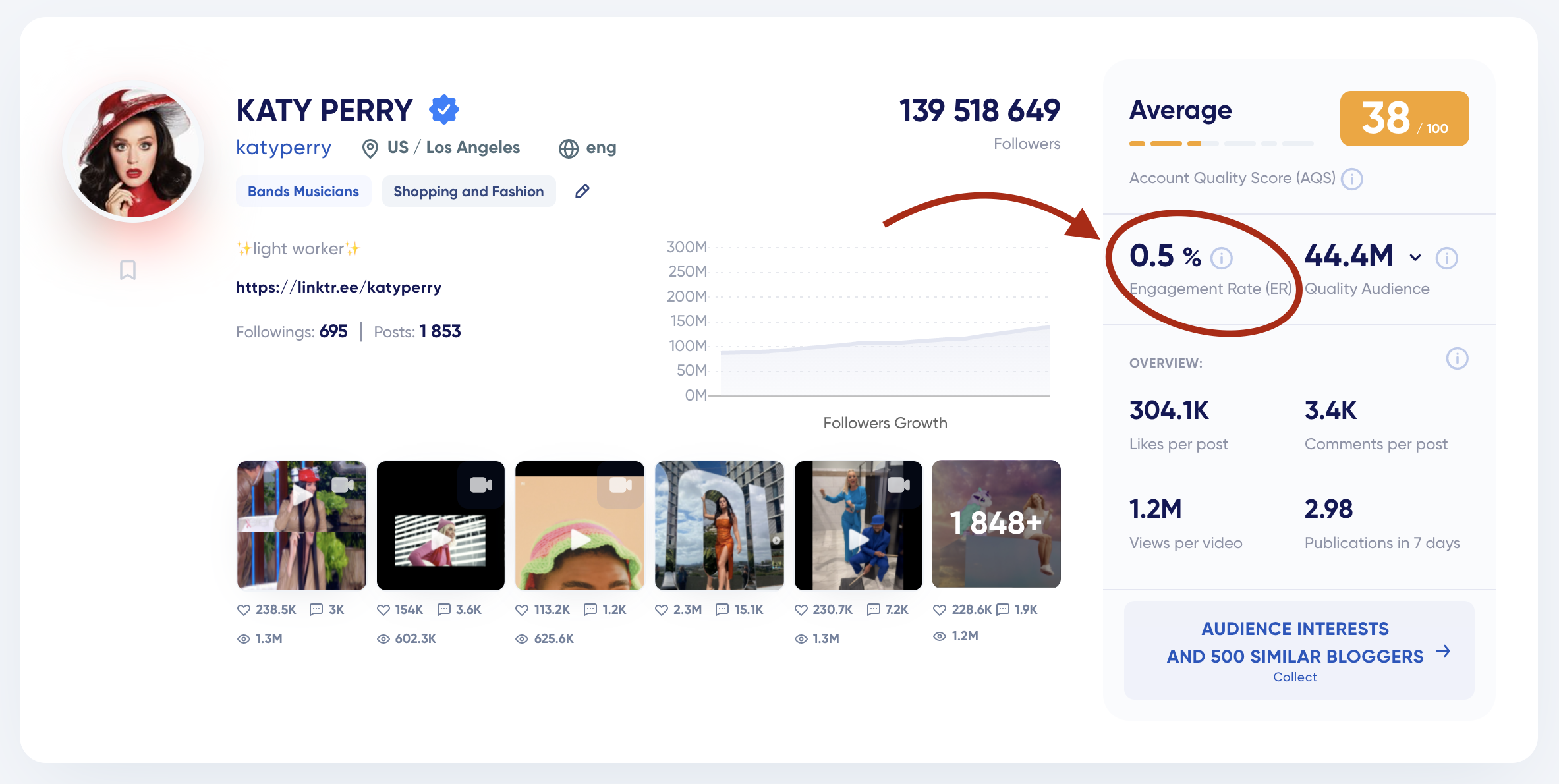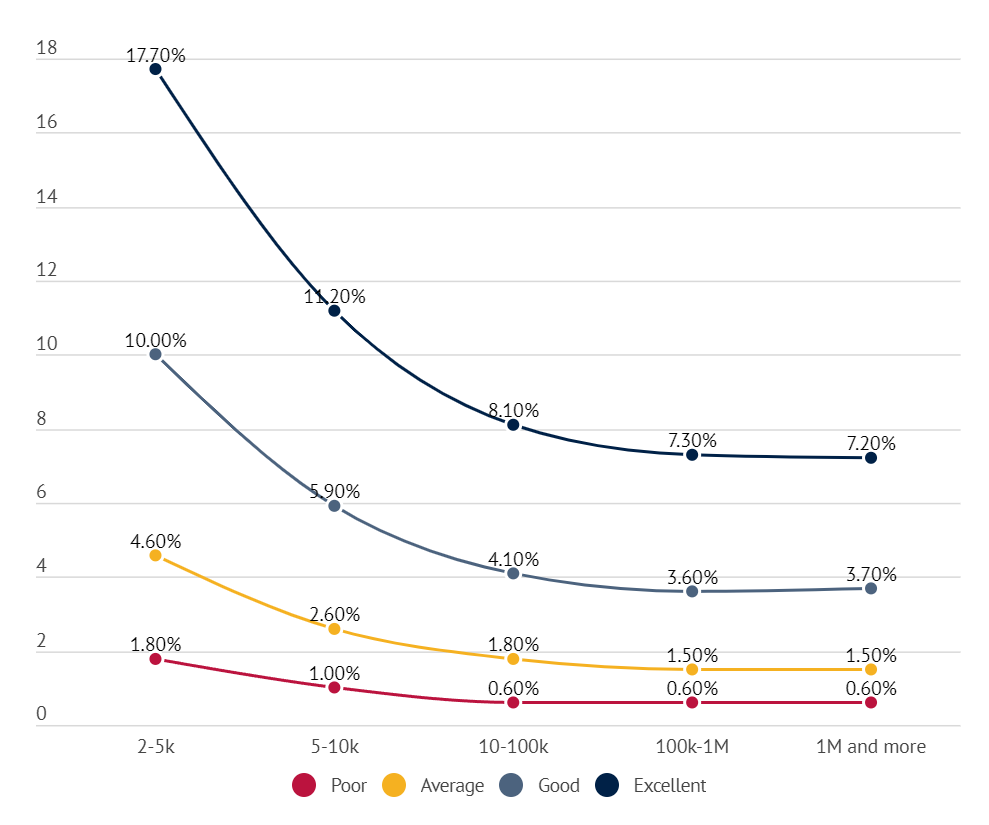# What is and how we calculate Engagement rateER (Engagement Rate) means the percentage of the audience that are engaged with the content, either commenting on or giving likes to the posts.

### How do we calculate it?

We look up the total likes and comments on the latest posts, divide the sum by the number of posts, and then we divide the result by the number of account followers.

For example, there are three posts in an account with 50k followers: the first one has 2,100 likes and 151 comments, the second one has 1,900 likes and 305 comments and the third one has 1,123 likes and 141 comments. In this case, the formula will look as follows:

((2,100 + 151 + 1,900 + 305 + 1,123 + 141) / 3 )/ 50,000 * 100% = 3.81%

### Excellent, Average and Poor ER for USA